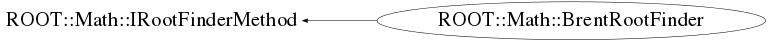# class ROOT::Math::BrentRootFinder: public ROOT::Math::IRootFinderMethod

```
Class for finding the root of a one dimensional function using the Brent algorithm.
It will use the Brent Method for finding function roots in a given interval.
First, a grid search is used to bracket the root value
with the a step size = (xmax-xmin)/npx. The step size
can be controlled via the SetNpx() function. A default value of npx = 100 is used.
The default value con be changed using the static method SetDefaultNpx.
If the function is unimodal or if its extrema are far apart, setting the fNpx to
a small value speeds the algorithm up many times.
Then, Brent's method is applied on the bracketed interval.
It will use the Brent Method for finding function roots in a given interval.
If the Brent method fails to converge the bracketing is repeted on the latest best estimate of the
interval. The procedure is repeted with a maximum value (default =10) which can be set for all
BrentRootFinder classes with the method SetDefaultNSearch

This class is implemented from TF1::GetX() method.

@ingroup RootFinders

```

## Function Members (Methods)

public:
 virtual ~BrentRootFinder() ROOT::Math::BrentRootFinder BrentRootFinder() ROOT::Math::BrentRootFinder BrentRootFinder(const ROOT::Math::BrentRootFinder&) ROOT::Math::IRootFinderMethod ROOT::Math::IRootFinderMethod::IRootFinderMethod() ROOT::Math::IRootFinderMethod ROOT::Math::IRootFinderMethod::IRootFinderMethod(const ROOT::Math::IRootFinderMethod&) virtual int ROOT::Math::IRootFinderMethod::Iterate() virtual int Iterations() const virtual const char* Name() const ROOT::Math::BrentRootFinder& operator=(const ROOT::Math::BrentRootFinder&) virtual double Root() const static void SetDefaultNpx(int npx) static void SetDefaultNSearch(int n) virtual bool SetFunction(const ROOT::Math::IGenFunction& f, double xlow, double xup) void SetLogScan(bool on) void SetNpx(int npx) virtual bool Solve(int maxIter = 100, double absTol = 1.0E-8, double relTol = 1.0E-10) virtual int Status() const

## Data Members

private:
 const ROOT::Math::IGenFunction* fFunction Pointer to the function. bool fLogScan flag to control usage of a log scan int fNIter Number of iterations needed for the last estimation. int fNpx Number of points to bracket root with initial grid (def is 100) double fRoot Current stimation of the function root. int fStatus Status of code of the last estimate double fXMax Upper bound of the search interval double fXMin Lower bound of the search interval.

## Class Charts## Function documentation

``` Default Constructor.
```
virtual ~BrentRootFinder()
``` Default Destructor.
```
`{}`
bool SetFunction(const ROOT::Math::IGenFunction& f, double xlow, double xup)
bool Solve(int maxIter = 100, double absTol = 1.0E-8, double relTol = 1.0E-10)
``` Returns the X value corresponding to the function value fy for (xmin<x<xmax).
Method:
First, the grid search is used to bracket the maximum
with the step size = (xmax-xmin)/fNpx. This way, the step size
can be controlled via the SetNpx() function. If the function is
unimodal or if its extrema are far apart, setting the fNpx to
a small value speeds the algorithm up many times.
Then, Brent's method is applied on the bracketed interval.

\@param maxIter maximum number of iterations.
\@param absTol desired absolute error in the minimum position.
\@param absTol desired relative error in the minimum position.

```
void SetNpx(int npx)
``` Set the number of point used to bracket root using a grid
```
`{ fNpx = npx; }`
void SetLogScan(bool on)
```          Set a log grid scan (default is equidistant bins)
will work only if xlow > 0

```
`{ fLogScan = on; }`
double Root() const
``` Returns root value. Need to call first Solve().
```
`{ return fRoot; }`
int Status() const
``` Returns status of last estimate. If = 0 is OK
```
`{ return fStatus; }`
int Iterations() const
``` Return number of iteration used to find minimum
```
`{ return fNIter; }`
const char* Name() const
``` Return name of root finder algorithm ("BrentRootFinder").
```
void SetDefaultNpx(int npx)
``` static function used to modify the default parameters
set number of default Npx used at construction time (when SetNpx is not called)
Default value is 100

```
void SetDefaultNSearch(int n)
``` set number of  times the bracketing search in combination with is done to find a good interval
Default value is 10

```